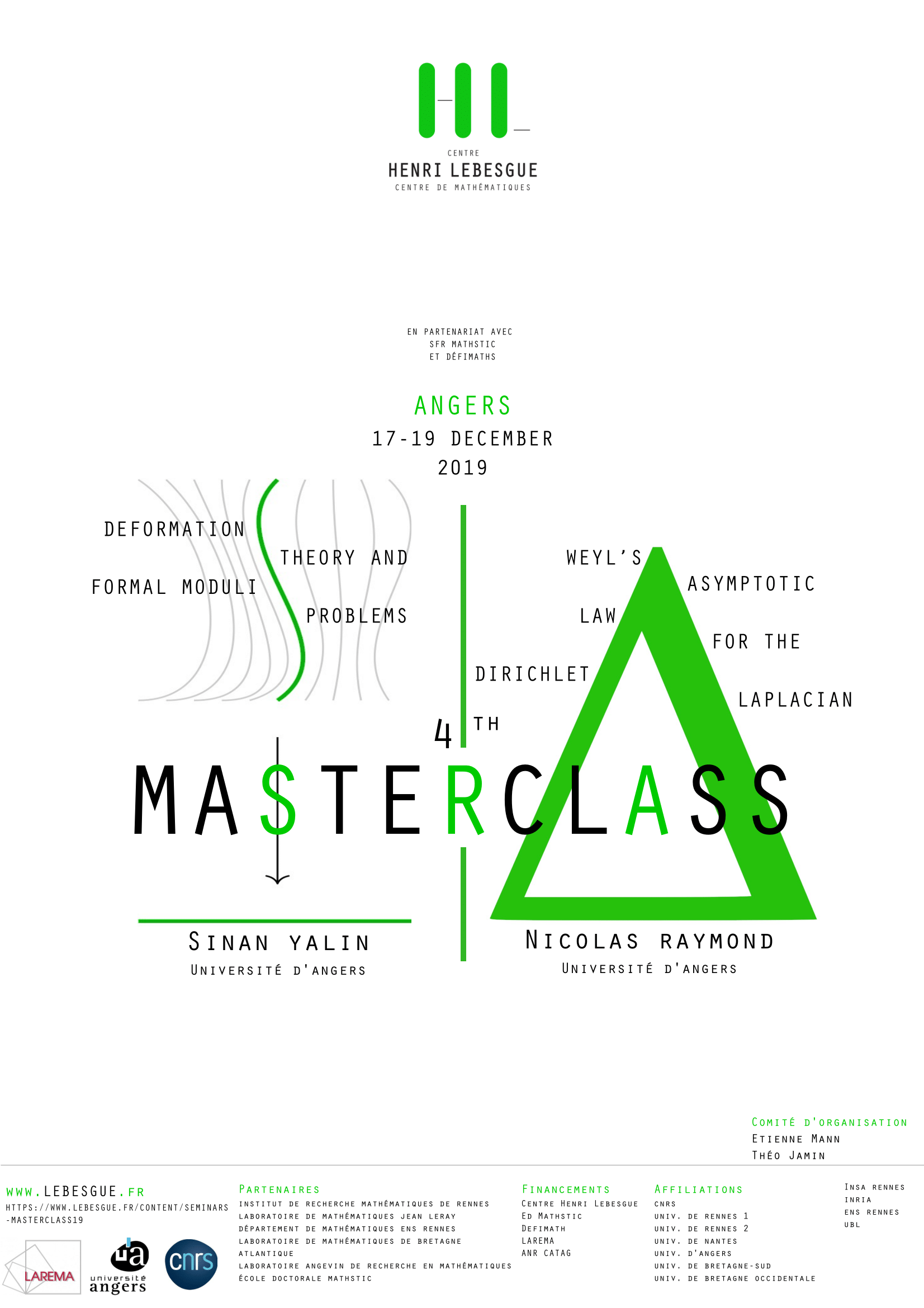# School - Masterclass 2019Angers, from december 17th to december 19th 2019

Organization board: Etienne Mann, Théo Jamin, Daniel Naie

We organize a masterclass in Angers, from 17th to 19th of December, 2019. The organisation board will pay the housing with breakfast and the lunches. The dinners are not payed by the organizers. For the travel expenses, we will do our best in the limit of our budget.

Registration are closed as we have reached the limit of participants.

Lectures will be in the morning sessions and exercises in the afternoon. We will have two parallel sessions:

• Sinan Yalin : Deformation theory and formal moduli problems
In this masterclass we will explain the formalism for encoding the infinitesimal deformation of structures that comes from algebra, topology or geometry. We will discuss some applications like quantification of Poisson structure by Kontsevich or some quantum invariants in topology of low dimension.
• Nicolas Raymond: Weyl's asymptotic law for the Dirichlet Laplacian
Notes of the lectures

This master class is devoted to the proof of the celebrated Weyl's asymptotic law. The aim is to estimate sum of eigenvalues of the Dirichlet Laplacian on a regular enough open set. The word "asymptotic" refers to the semiclassical limit $h\to 0$. More precisely, one will show that

[\begin{align*}\mathrm{Tr}(-h^2\Delta-1)-=&L_d|\Omega|h^{-d}-\frac{1}{4}L{d-1}|\partial\Omega|h^{-d+1}\&+o(h^{-d+1})\end{align*}] where [L_d=(2\pi)^{-d}\int_{\mathbb{R}^d}(\xi^2-1)_-\mathrm{d},\xi,.] The meaning of the symbol $\mathrm{Tr}$ will be explained in the first part of the lecture through the theory of trace-class and Hilbert-Schmidt operators. In the second part, we will prove the asymptotic formula by means of "standard" semiclassical tools. This second part can be seen as a lecture/exeresis on the following article:
R. L. Frank and L. Geisinger. Two-term spectral asymptotics for the Dirichlet Laplacian on a bounded domain. In Mathematical results in quantum physics, pages 138--147. World Sci. Publ., Hackensack, NJ, 2011.